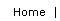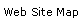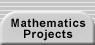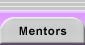It is sometimes difficult (or impossible) to prove that a conjecture is true using direct methods. For example, to show that the square root of two is irrational, we cannot directly test and reject the infinite number of rational numbers whose square might be two. Instead, we show that the assumption that root two is rational leads to a contradiction. The steps taken for a proof by contradiction (also called indirect proof) are:

1. Assume the opposite of your conclusion.
1. For “the primes are infinite in number,” assume that the primes are a finite set of size n.
2. To prove the statement “if a triangle is scalene, then no two of its angles are congruent,” assume that at least two angles are congruent.
2. Use the assumption to derive new consequences until one is the opposite of your premise. For the two examples above, you would seek to establish:
1. that there exists a prime not counted in the initial set of n primes.
2. that the triangle cannot be scalene.
3. Conclude that the assumption must be false and that its opposite (your original conclusion) must be true.

Why does this method make sense? One way to understand it is to note that you are creating a direct proof of the contrapositive of your original statement (you are proving if not B, then not A). Because contrapositive statements are always logically equivalent, the original then follows.

Note that the contradiction forces us to reject our assumption because our other steps based on that assumption are logical and justified. The only “mistake”that we could have made was the assumption itself. An indirect proof establishes that the opposite conclusion is not consistent with the premise and that, therefore, the original conclusion must be true.

### Opposites

With the Republicans in power, it’s man eat man. With the Democrats, it’s just the opposite. — Bumper sticker.

Sometimes, it can be a challenge determining what the opposite of a conclusion is. The opposite of “all X are Y” is not “all X are not Y,” but “at least one X is not Y.” Similarly, when we have a compound conclusion, we need to be careful. Consider these two examples:

 The Original Conjecture The Opposite of the Conclusion If m and n are integers and mn is odd, then m is odd and n is odd. m is even or n is even If m + n is irrational, then m is irrational or n is irrational. m is rational and n is rational

### Resources

See Triangle with Restricted Angle Sum for a practice problem and Proof by Contradiction Class Activity for a lesson plan that introduces proof by contradiction.Translations of mathematical formulas for web display were created by tex4ht. © Copyright 2003 Education Development Center, Inc. (EDC)